Function Repository Resource:

Contributed by: Bob Sandheinrich

## Examples

### Basic Examples (2)

 In:=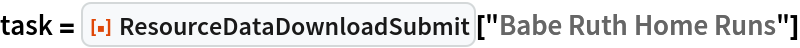Out=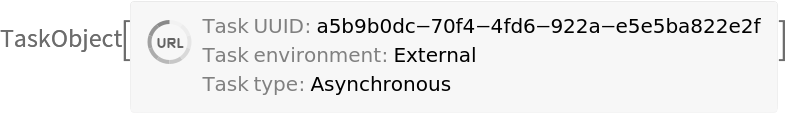When the task is complete, the data is immediately available via ResourceData:

 In:=Out=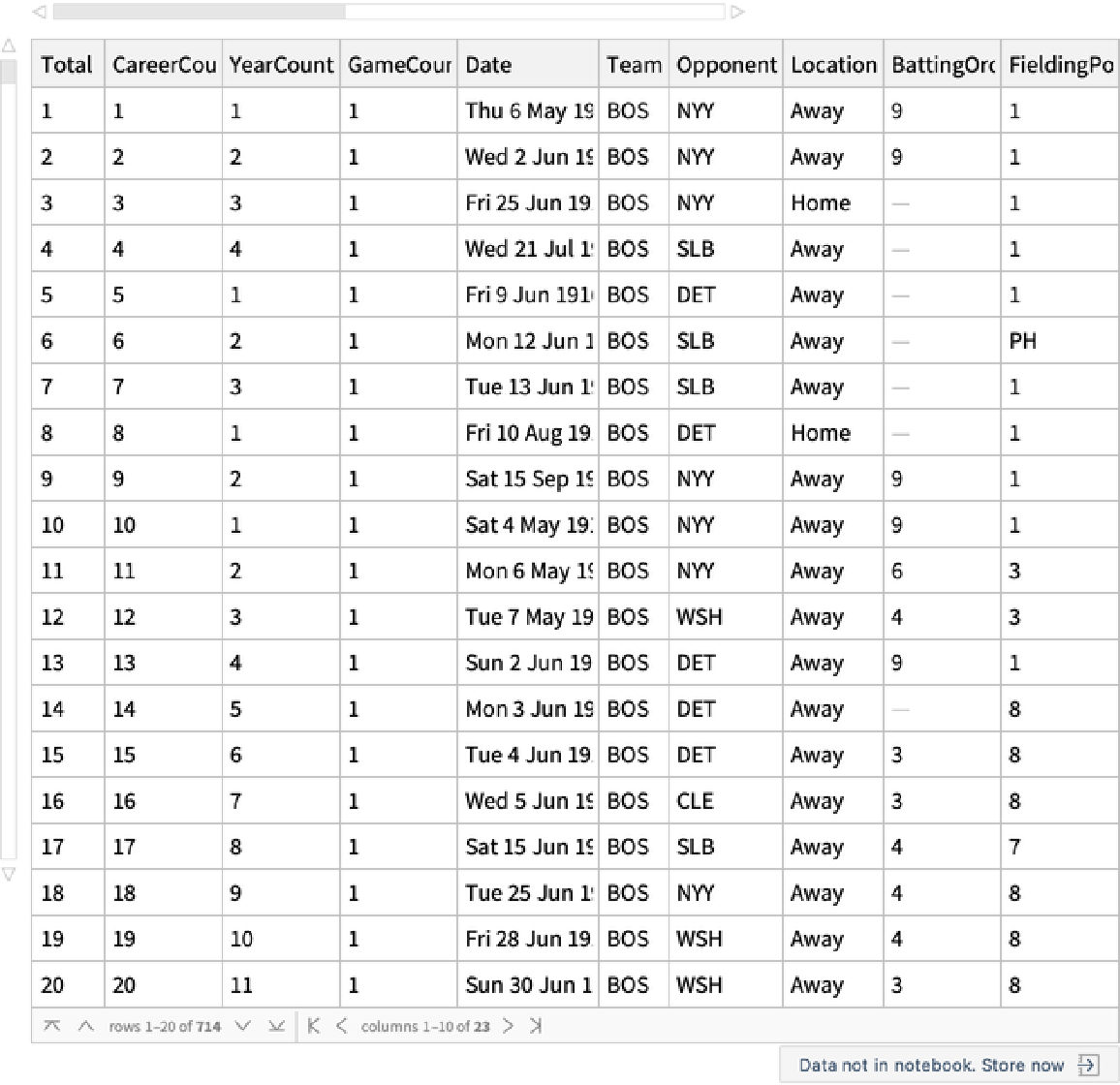In:=Out=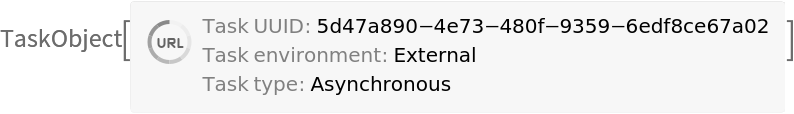When the task is complete, the neural network is immediately available via NetModel:

 In:=Out=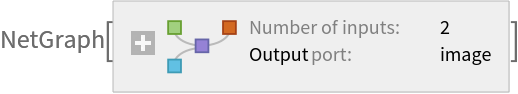### Scope (3)

 In:=Out=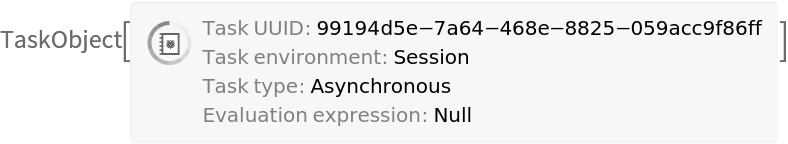In:=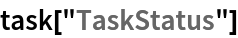Out=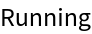In:=Out=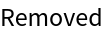The data is now stored locally:

 In:=Out=Importing the data is now fast:

 In:=Out=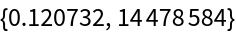In:=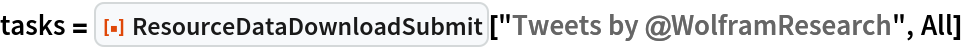Out=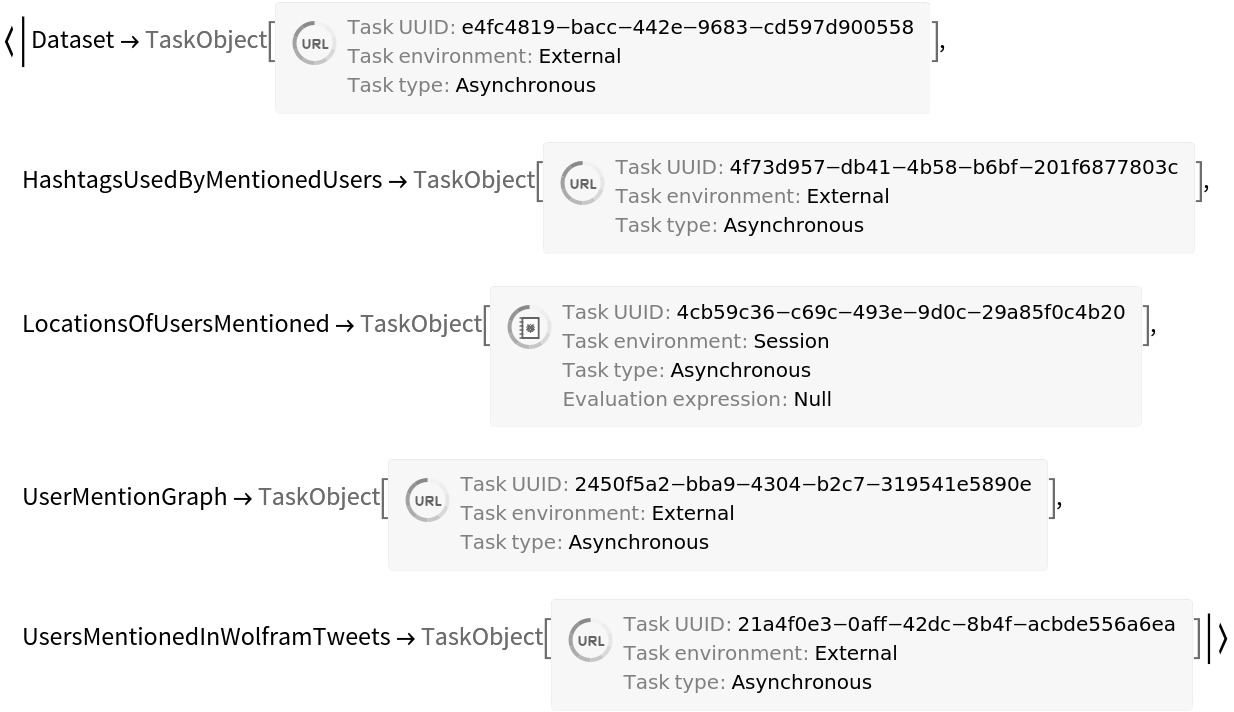In:=Out=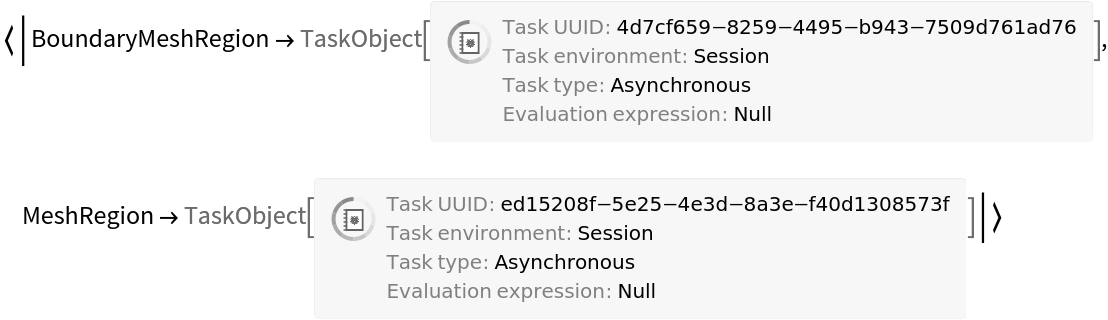Bob

## Version History

• 1.0.0 – 29 March 2022